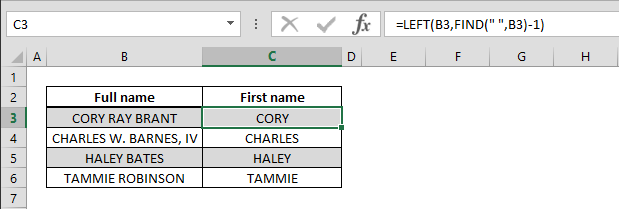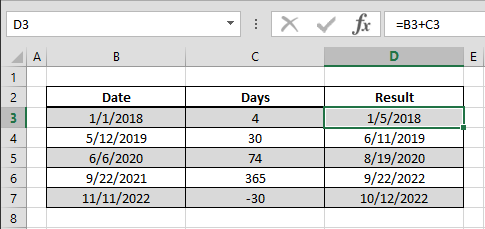Select Page## How to find the power of a number

You can always use caret symbol (^) to find a certain power of a number. However, doing this with a formula can be easier to read and identify in nested formula sets. Syntax =POWER(base number, how many times that base number will be multiplied by itself) Steps Begin...## How to get the column index of a cell using the COLUMN function

Working with Excel’s coordinate system makes building data models extremely easy. You can retrieve the column index information of a cell using the COLUMN function and implement in other formulas. Syntax =COLUMN(cell or range reference you want get its column...## How to get the row index of a cell using the ROW function

Working with Excel’s coordinate system makes building data models extremely easy. You can retrieve the row index information of a cell using the ROW function and implement in other formulas. Syntax =ROW(cell or range reference you want get its row number) Steps...## How to get the first name text from the full name data

One perfect example of automatically cleaning up data in Excel is when working with name fields. You can separate first and last names (or actually any text with spaces) using this method. Syntax =LEFT(Full name, FIND(” “, Full name) – 1) Steps Begin by...## How to search for a string or check if cell contains a specific text

Excel’s Search and Find feature is very helpful when looking up things in workbooks, but this means searching for keywords one by one. SEARCH and FIND are formula versions of this very handy feature and can work wonders in large data sets. Syntax...## How to use day information from date data in Excel

Working with dates in Excel can be a bit challenging at times, because Excel doesn’t use dates as we conceive it. Knowing how Excel handles dates, you will find it pretty easy to use them in calculations and reports. Syntax =date + days Steps Select or type in...## How to SUM cells by an equal criteria

Do you have a data table where you need to pick all the highest, lowest, best, worst, or equal to 2? SUMIF, and its bigger brother SUMIFS formulas can help sum cells of your choice. Syntax =SUMIF(criteria range, criteria, values to sum range) =SUMIFS(values to sum...